Comparison
Comparison close
Results

CCQM-K17 and EUROMET.QM-K17

MEASURAND : pH value of phthalate buffer
Sample: Potassium hydrogen phthalate, KHC8H4O4
Measurements at 15 °C
NOMINAL VALUE : pH = 4.0 at 25 °C

Degrees of equivalence Di and expanded uncertainty Ui (k = 2)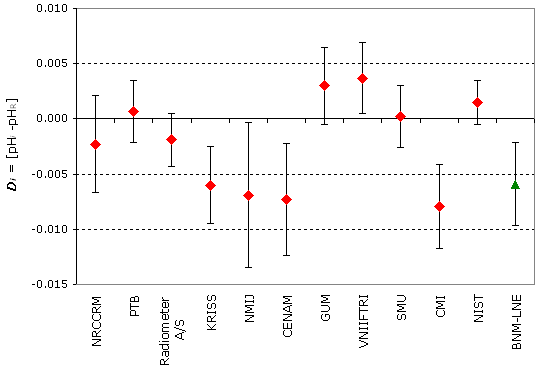Red diamonds: participants in CCQM-K17
Green triangle: participant in EUROMET.QM-K17

CCQM-K17 and EUROMET.QM-K17

MEASURAND : pH value of phthalate buffer
Sample: Potassium hydrogen phthalate, KHC8H4O4
Measurements at 25 °C
NOMINAL VALUE : pH = 4.0 at 25 °C

Degrees of equivalence Di and expanded uncertainty Ui (k = 2)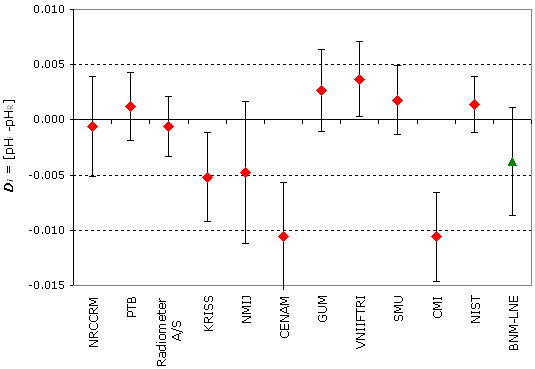Red diamonds: participants in CCQM-K17
Green triangle: participant in EUROMET.QM-K17

CCQM-K17 and EUROMET.QM-K17

MEASURAND : pH value of phthalate buffer
Sample: Potassium hydrogen phthalate, KHC8H4O4
Measurements at 37 °C
NOMINAL VALUE : pH = 4.0 at 25 °C

Degrees of equivalence Di and expanded uncertainty Ui (k = 2)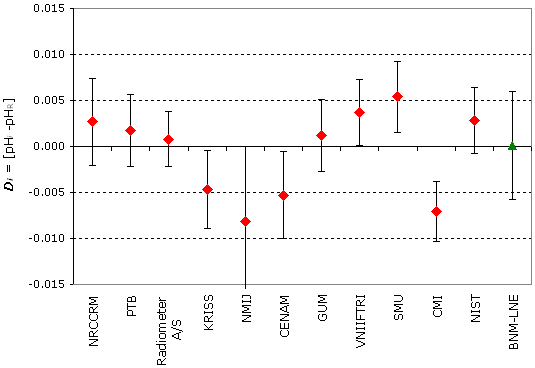Red diamonds: participants in CCQM-K17
Green triangle: participant in EUROMET.QM-K17

Comparison
Comparison close
Results

CCQM-K17 and EUROMET.QM-K17

MEASURAND : pH value of phthalate buffer
Sample: Potassium hydrogen phthalate, KHC8H4O4
Measurements at 15 °C
NOMINAL VALUE : pH = 4.0 at 25 °C

Degrees of equivalence Di and expanded uncertainty Ui (k = 2)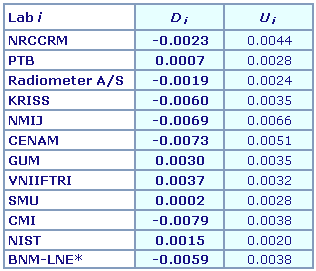BNM-LNE*: participant in EUROMET.QM-K17

CCQM-K17 and EUROMET.QM-K17

MEASURAND : pH value of phthalate buffer
Sample: Potassium hydrogen phthalate, KHC8H4O4
Measurements at 25 °C
NOMINAL VALUE : pH = 4.0 at 25 °C

Degrees of equivalence Di and expanded uncertainty Ui (k = 2)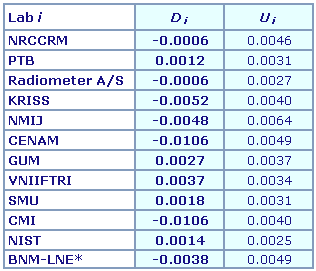BNM-LNE*: participant in EUROMET.QM-K17

CCQM-K17 and EUROMET.QM-K17

MEASURAND : pH value of phthalate buffer
Sample: Potassium hydrogen phthalate, KHC8H4O4
Measurements at 37 °C
NOMINAL VALUE : pH = 4.0 at 25 °C

Degrees of equivalence Di and expanded uncertainty Ui (k = 2)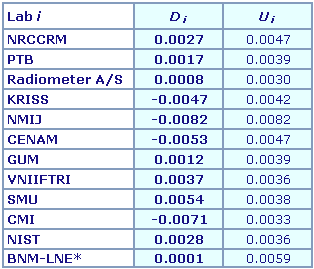BNM-LNE*: participant in EUROMET.QM-K17

Comparison
Comparison close
CC comparison
 Metrology area, Sub-field Chemistry and Biology, Electrochemistry Description pH measurement of phthalate buffer solutions Time of measurements 2003 Status Approved for equivalence
 Final Reports of the comparisons Measurand pH 4.1 at temperature 25 °C Parameters Temperature: 15 °C, 25 °C and 37 °C Transfer device A phthalate buffer, containing potassium hydrogen phthalate at a molality of 0.05 mol/kg
 Comparison type Key Comparison Consultative Committee CCQM (Consultative Committee for Amount of Substance) Conducted by EURAMET (European Association of National Metrology Institutes) RMO Internal Identifier EUROMET Project No 696 Comments EUROMET.QM-K17 results are linked to those of CCQM-K17. CCQM-K17 results were published on 8 September 2003, and EUROMET.QM-K17 results on 28 February 2005. pH determination of a phthalate buffer at temperatures ranging from 15 °C to 37 °C. Pilot institute PTB Physikalisch-Technische Bundesanstalt Germany Contact person P. Spitzer +49 (0) 531 592 3130
First Name Last Name
wwww@ww.www +356719836 Institute 1 Institute 1 Khmelnitskiy
Pilot laboratory
PTB

Physikalisch-Technische Bundesanstalt, Germany, EURAMET

 BNM-LNE Bureau National de Métrologie - Laboratoire National d'Essais, France, EURAMET
 VNIIFTRI Institute of Physical Technical and Radiotechnical Measurements, Rosstandart, Russian Federation, COOMET
Comparison
Comparison close
Results

CCQM-K17 and EUROMET.QM-K17

MEASURAND : pH value of phthalate buffer
Sample: Potassium hydrogen phthalate, KHC8H4O4
Measurements at 15 °C
NOMINAL VALUE : pH = 4.0 at 25 °C

• Key comparison CCQM-K17

 The key comparison reference value, pHR, is obtained as the variance-weighted mean of the results from the participants. The standard uncertainty, uR, of pHR is calculated according to the external consistency concept (see page 8 of CCQM-K17 Final Report). pHR = 4.0093 and uR = 0.00089
 The degree of equivalence of each laboratory with respect to the key comparison reference value is given by a pair of terms: Di = (pHi - pHR) and Ui, its expanded uncertainty (k = 2), Ui = 2(ui2 + uR2)1/2. Note that this gives a conservative estimate of Ui.

 The degree of equivalence between two laboratories may be calculated using the following pair of terms: Dij = Di - Dj = pHi - pHj and Uij, its expanded uncertainty (k = 2), Uij = 2(ui2 + uj2)1/2.No numerical values of pair-wise degrees of equivalence are given.

The PTB acted as pilot laboratory in both key comparisons.
To link the results obtained by the BNM-LNE in EUROMET.QM-K17 to CCQM-K17 the performance of PTB within its uncertainty is assumed to be similar in both comparisons. Therefore it is assumed that the bias between the PTB results and the key comparison reference value remains constant over both comparisons.

 The degree of equivalence of the BNM-LNE with respect to the key comparison reference value is given by a pair of terms: Di and Ui, its expanded uncertainty(k = 2), see equations 1 to 4 in the EUROMET.QM-K17 Final Report.

 The pair-wise degrees of equivalence involving the BNM-LNE are not calculated.

CCQM-K17 and EUROMET.QM-K17

MEASURAND : pH value of phthalate buffer
Sample: Potassium hydrogen phthalate, KHC8H4O4
Measurements at 25 °C
NOMINAL VALUE : pH = 4.0 at 25 °C

• Key comparison CCQM-K17

 The key comparison reference value, pHR, is obtained as the variance-weighted mean of the results from the participants. The standard uncertainty, uR, of pHR is calculated according to the external consistency concept (see page 8 of CCQM-K17 Final Report). pHR = 4.0156 and uR = 0.00109
 The degree of equivalence of each laboratory with respect to the key comparison reference value is given by a pair of terms: Di = (pHi - pHR) and Ui, its expanded uncertainty (k = 2), Ui = 2(ui2 + uR2)1/2. Note that this gives a conservative estimate of Ui.

 The degree of equivalence between two laboratories may be calculated using the following pair of terms: Dij = Di - Dj = pHi - pHj and Uij, its expanded uncertainty (k = 2), Uij = 2(ui2 + uj2)1/2.No numerical values of pair-wise degrees of equivalence are given.

The PTB acted as pilot laboratory in both key comparisons.
To link the results obtained by the BNM-LNE in EUROMET.QM-K17 to CCQM-K17 the performance of PTB within its uncertainty is assumed to be similar in both comparisons. Therefore it is assumed that the bias between the PTB results and the key comparison reference value remains constant over both comparisons.

 The degree of equivalence of the BNM-LNE with respect to the key comparison reference value is given by a pair of terms: Di and Ui, its expanded uncertainty(k = 2), see equations 1 to 4 in the EUROMET.QM-K17 Final Report.

 The pair-wise degrees of equivalence involving the BNM-LNE are not calculated.

CCQM-K17 and EUROMET.QM-K17

MEASURAND : pH value of phthalate buffer
Sample: Potassium hydrogen phthalate, KHC8H4O4
Measurements at 37 °C
NOMINAL VALUE : pH = 4.0 at 25 °C

• Key comparison CCQM-K17

 The key comparison reference value, pHR, is obtained as the variance-weighted mean of the results from the participants. The standard uncertainty, uR, of pHR is calculated according to the external consistency concept (see page 8 of CCQM-K17 Final Report). pHR = 4.0343 and uR = 0.00125
 The degree of equivalence of each laboratory with respect to the key comparison reference value is given by a pair of terms: Di = (pHi - pHR) and Ui, its expanded uncertainty (k = 2), Ui = 2(ui2 + uR2)1/2. Note that this gives a conservative estimate of Ui.

 The degree of equivalence between two laboratories may be calculated using the following pair of terms: Dij = Di - Dj = pHi - pHj and Uij, its expanded uncertainty (k = 2), Uij = 2(ui2 + uj2)1/2.No numerical values of pair-wise degrees of equivalence are given.

The PTB acted as pilot laboratory in both key comparisons.
To link the results obtained by the BNM-LNE in EUROMET.QM-K17 to CCQM-K17 the performance of PTB within its uncertainty is assumed to be similar in both comparisons. Therefore it is assumed that the bias between the PTB results and the key comparison reference value remains constant over both comparisons.

 The degree of equivalence of the BNM-LNE with respect to the key comparison reference value is given by a pair of terms: Di and Ui, its expanded uncertainty (k = 2), see equations 1 to 4 in the EUROMET.QM-K17 Final Report.

 The pair-wise degrees of equivalence involving the BNM-LNE are not calculated.

Comparison
Comparison close
Results

CCQM-K17 and EUROMET.QM-K17

MEASURAND : pH value of phthalate buffer
Sample: Potassium hydrogen phthalate, KHC8H4O4
Measurements at 15 °C
NOMINAL VALUE : pH = 4.0 at 25 °C

pHi : result of measurement carried out by laboratory i
ui : combined standard uncertainty (k = 1) of pHi
Ui : expanded uncertainty (k = 2) of pHi, Ui = 2ui

• Key comparison CCQM-K17

 Lab i pHi ui Date ofmeasurement NRCCRM 4.0070 0.0020 Aug 01 PTB 4.0100 0.0011 Jul 01 Radiometer A/S 4.0074 0.0008 Jul 01 KRISS 4.0033 0.0015 Jul 01 NMIJ 4.0024 0.0032 Jul 01 CENAM 4.0020 0.0024 Jul 01 GUM 4.0123 0.0015 Jul 01 VNIIFTRI 4.0130 0.0013 Jul 01 SMU 4.0095 0.0011 Sep 01 CMI 4.0014 0.0017 Sep 01 NIST 4.0108 0.0005 Jul 01

• Key comparison EUROMET.QM-K17

 Lab i pHi Ui Date ofmeasurement BNM-LNE 3.9919 0.0024 Feb 03 PTB 3.9985 0.0024 Jan 03

CCQM-K17 and EUROMET.QM-K17

MEASURAND : pH value of phthalate buffer
Sample: Potassium hydrogen phthalate, KHC8H4O4
Measurements at 25 °C
NOMINAL VALUE : pH = 4.0 at 25 °C

pHi : result of measurement carried out by laboratory i
ui : combined standard uncertainty (k = 1) of pHi
Ui : expanded uncertainty (k = 2) of pHi, Ui = 2ui

• Key comparison CCQM-K17

 Lab i pHi ui Date of measurement NRCCRM 4.0150 0.0020 Aug 01 PTB 4.0168 0.0011 Jul 01 Radiometer A/S 4.0150 0.0008 Jul 01 KRISS 4.0104 0.0017 Jul 01 NMIJ 4.0108 0.0030 Jul 01 CENAM 4.0050 0.0022 Jul 01 GUM 4.0183 0.0015 Jul 01 VNIIFTRI 4.0193 0.0013 Jul 01 SMU 4.0174 0.0011 Sep 01 CMI 4.0050 0.0017 Sep 01 NIST 4.0170 0.0006 Jul 01

• Key comparison EUROMET.QM-K17

 Lab i pHi Ui Date ofmeasurement BNM-LNE 4.0002 0.0038 Feb 03 PTB 4.0052 0.0024 Jan 03

CCQM-K17 and EUROMET.QM-K17

MEASURAND : pH value of phthalate buffer
Sample: Potassium hydrogen phthalate, KHC8H4O4
Measurements at 37 °C

NOMINAL VALUE : pH = 4.0 at 25 °C

pHi : result of measurement carried out by laboratory i
ui : combined standard uncertainty (k = 1) of pHi
Ui : expanded uncertainty (k = 2) of pHi, Ui = 2ui

• Key comparison CCQM-K17

 Lab i pHi ui Date of measurement NRCCRM 4.0370 0.0020 Aug 01 PTB 4.0360 0.0015 Jul 01 Radiometer A/S 4.0351 0.0008 Jul 01 KRISS 4.0296 0.0017 Jul 01 NMIJ 4.0261 0.0039 Jul 01 CENAM 4.0290 0.0020 Jul 01 GUM 4.0355 0.0015 Jul 01 VNIIFTRI 4.0380 0.0013 Jul 01 SMU 4.0397 0.0015 Sep 01 CMI 4.0272 0.0011 Sep 01 NIST 4.0371 0.0013 Jul 01

• Key comparison EUROMET.QM-K17

 Lab i pHi Ui Date ofmeasurement BNM-LNE 4.0209 0.0046 Feb 03 PTB 4.0225 0.0024 Jan 03